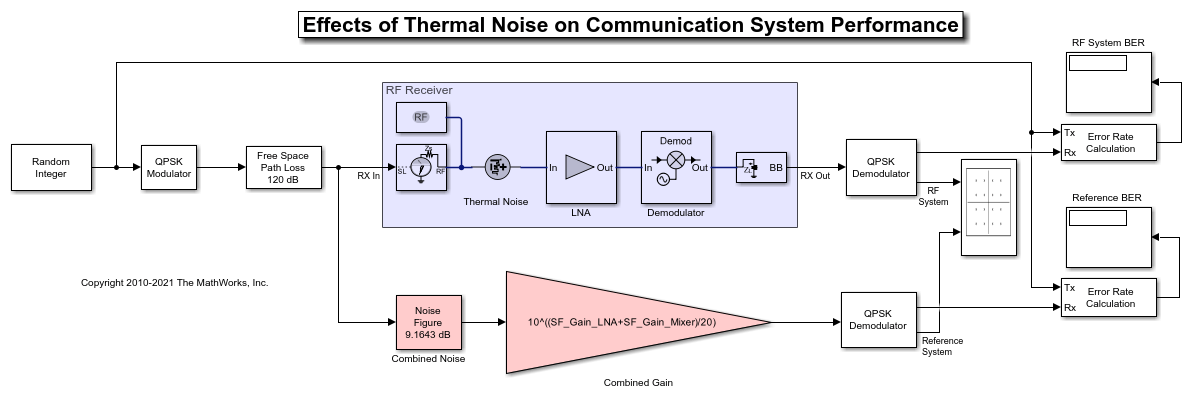# Impact of Thermal Noise on Communication System Performance

This example shows how to use the RF Blockset Circuit Envelope library to model the thermal noise of a super-heterodyne RF receiver and measure its effects on the bit error rate (BER) of a communications system. The RF receiver model does not include impedance mismatches or nonlinearities. A Communications Toolbox reference model with parameters computed using Friis equations is used to verify the results.

The Modulator and Channel subsystems consist of blocks that model:

• A QPSK-modulated waveform of random bits

• A raised cosine pulse-shaping filter for spectral limiting

• Free-space path loss

The RF receiver subsystem, shown in the light purple area, consists of:

• An Inport block, which assigns the complex input waveform to the specified RF carrier. In this case the variable SF_RF is set to 2.1 GHz. To ensure that the RF Blockset model has the same available power referred to a 50 Ohm impedance as the reference model, the Source type parameter is set to Power.

• A cascaded RF amplifier and RF demodulator with specified noise figure and gain. The amplifier and the mixer do not specify any nonlinearity, so the harmonic order of the simulation specified in the configuration block is set to 1.

• An Outport block, with the Source type parameter set to Power and center frequency equal to the intermediate frequency given by the absolute difference between the RF center frequency specified in the Inport and Local Oscillator specified in the demodulator. In this case, the intermediate frequency is equal to the variable SF_IF = 500 MHz.

• A thermal white noise source at the input of the chain to model the equivalent noise floor introduced by an ideally matched antenna (50 Ohm) at 290 K.

• An image reject filter is added into the Demodulator block using a mask checkbox to ensure that only signals centered around the 2.1 GHz carrier enter the demodulator. This filter also prevents the down-conversion of thermal noise centered around the image frequency of 2.6 GHz (image frequency is SL_RF+SL_IF) into the intermediate frequency. If the image rejection filter is removed, the noise contribution on the output signal increases above the estimation provided by Friis equations and the BER will deteriorate.

• Note that all blocks in the RF receiver are ideally matched to 50 Ohm. To understand the effects of impedance mismatches on noise simulation see the example RF Noise Modeling.

The reference system, shown in red, consists of:

• A Receiver Thermal Noise block that adds noise to the signal according to the value calculated by the Friis Equation. You can find the calculation in the model's pre-load callback function. This block also includes the equivalent thermal noise introduced by the antenna.

• A Gain block that models the combined gain of the RF receiver.

• Baseband filters and demodulators process the received signal.

### Circuit Envelope Simulation of the RF Receiver

 open_system('RFReceiverImpactExample')Select Simulation > Run.

Error Rate Calculation blocks compute the BER for the system and reference. To observe the BER as it approaches steady state, increase the total simulation time. For this example, the steady-state bit error rate is approximately 1e-4.

### Computing RF Receiver Noise Figure

To model noise in the RF Blockset circuit envelope environment:

• In the Configuration block dialog, select Simulate noise.

• Specify the Noise figure (dB) parameter of RF Amplifier and RF Mixer blocks in your system. The following specifications for the RF receiver in this example produce a combined noise figure of 9.16 dB (as per the Friis Equation): LNA gain of 20 dB, LNA noise figure of 9 dB, and RF mixer noise figure of 15 dB.bdclose('RFReceiverImpactExample')